[Introduction]  [Signal arithmetic]  [Signals and noise]   [Smoothing]   [Differentiation]  [Peak Sharpening]  [Harmonic analysis]   [Fourier convolution]  [Fourier deconvolution]  [Fourier filter]  [Wavelets]  [Peak area measurement]  [Linear Least Squares]  [Multicomponent Spectroscopy]  [Iterative Curve Fitting]  [Hyperlinear quantitative absorption spectrophotometry] [Appendix and Case Studies]  [Peak Finding and Measurement]  [iPeak]   [iSignal]  [Peak Fitters]   [iFilter]  [iPower]  [List of downloadable software]  [Interactive tools]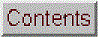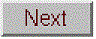## Wavelets and wavelet denoising

[Visualization and analysis]   [Wavelet denoising]

Wavelets are literally “little waves”, small oscillating waveforms that begin from zero, swell to a maximum, and then quickly decay to zero again. They can be contrasted to, for example, sine or cosine waves, which go on “forever”, repeating out to positive and negative infinity. In the previous sections we have seen how useful it is to use the Fourier Transform of a signal, which expresses a signal as the sum of sine and cosine waves, allowing such useful operations as convolution, deconvolution, and Fourier filtering. But there is a down side to the Fourier Transform; it covers the entire signal duration, giving only the average frequency content. We saw previously that it is possible to use segmented or time-resolved variations of the Fourier transform to overcome this difficulty. But a more sophisticated way to solve this limitation of Fourier analysis is to use wavelets as a basis set for representing signals rather than sine and cosine waves. Like sine waves, wavelets can be stretched or compressed along their “x” or time axis to cover different frequencies. But unlike sine waves, wavelets can be translated along the time axis of a signal to probe the time variations, because wavelets are of short duration compared with the signals they are used with.

Wavelets were introduced by mathematicians and mathematical physicists in the early years of the 20th century and the subsequent development has been highly mathematical. Many of the treatments of wavelets in the literature are aimed at the mathematical aspects, which have beenworked out in excruciating detail” (reference 80). The value system of mathematics – rigorous proofs, exhaustive exploration, assumption of mathematical background, and the need for compact notation - make it difficult for the non-specialists. Because of this, there are an unusually large number of “easy” introductions to the subject (references 77 - 80) that promise to soften the blow of mathematical abstraction. For that reason, I will not repeat the mathematical details here. Rather, I will attempt to show what you can accomplish using wavelets without understanding all the underlying mathematics. I am particularly interested in situations when the wavelets works better than the best available conventional techniques, but also in situations where the conventional techniques remain superior.

A wavelet transform (WT) is a decomposition of a signal into a set of basis functions consisting of contractions, expansions, and translations of a wavelet function (reference 83). It can be computed by repeated convolution of the signal with the chosen wavelet as the wavelet is translated across the time dimension, in order to probe the time variation, and as the wavelet is stretched or compressed, in order to probe difference frequencies. Because two dimensions are being probed, the result is naturally a 3D surface (time-frequency-amplitude) that can be conveniently displayed as a time-frequency contour plot with different colors representing the amplitudes at that time and frequency. Of course, one expects that such calculations will require more complex algorithms and greater execution times. That might have been a problem in the early days of computers, but with modern fast processors and great memory capacity, it’s unlikely to be of concern.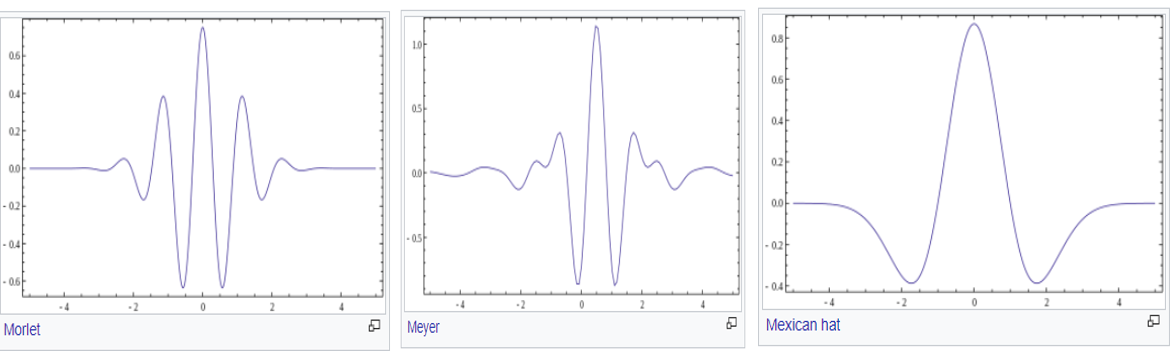Wavelets are used for the visualization, analysis, compression, and denoising of complex data. There are dozens of different wavelet shapes, which by itself is a big difference from Fourier analysis. Three of them, the Meyer, the Morlet and the Mexican hat, are mentioned and pictured in the Wikipedia article on wavelets.

In Matlab, the easiest way to access these tools is to use the Wavelet Toolbox, if that is included in your school or company campus Matlab site license. This toolbox includes a graphical user interface (GUI) for a Wavelet Analyzer, Signal Multiresolution Analyzer, and a Wavelet Signal Denoiser, as well as an extensive collection of command-line wavelet functions. Documentation is available at https://www.mathworks.com/products/wavelet.html. It’s not absolutely necessary to have the Wavelet Toolbox, however. Plenty of code has been published on the Internet in a variety of languages. For example, Michael Cohen’s paper (reference 82) includes Matlab code that implements a Morlet wavelet using only the inbuilt functions fft.m, ifft.m, and conv.m. I will use all of these software approaches to describe the properties and applications of wavelets to scientific measurement.

### Visualization and analysis

Wavelets are quite effective as visualizing complicated signals and helping the scientist make sense of them. A good example is given in reference 82, which describes a complex signal sampled at 1000 Hz and consisting of three overlapping components that are initially unknown to the experimenter. The separate components are shown in the figure below: 1) a swept sine wave (‘chirp’) going from 5 Hz to 20 Hz, (2) another simultaneous swept sine wave (‘chirp’) going in reverse, from 20 Hz to 5 Hz, and finally (3) a Gaussian-modulated 20 Hz sine wave that peaks in the center of the signal.

When there three added are up, the resulting time-domain waveform, shown in the upper panel of the figure below (displayed in iSignal.m), is complicated and offers no hint of its underlying structure. The conventional Fourier transform spectrum, shown in the lower panel, offers no help. In fact, the Fourier spectrum is misleading; it suggests that there might be only two components, one at a higher frequency range than the other, with a small gap in between near 12 Hz. But in fact there are actually three components, two of them covering a wide frequency range and the third one fixed at about 12 Hz.

In contrast, the wavelet-based time-frequency-amplitude contour plot shown below, which is computed by the Matlab code in the original paper, helps to unravel the complexities, showing all three components. In this display, yellow corresponds to the greatest amplitudes and blue to the lowest.

(There is an ambiguity concerning the two swept sine waves at the point where they cross in frequency in the middle of the signal; do they keep going in the same direction, forming an “X”, or do they both reverse direction, forming a “V” and its reflection?  The two behaviors would result in the same final signal. The simplest assumption would be the former).

Another example is based on the “buried peaks” signal that has been used before. This example is closer to conventional scientific application: digging peaks in a signal out of an excess of noise and interference. The signal (top panel in the figure below) consists of a pair of weak Gaussian peaks that are completely buried in a much stronger interfering swept-frequency sine wave and random white noise. The Fourier spectrum, observed here in the bottom panel, again offers little hint of the underlying structure.

But the Morlet wavelet can be applied to this signal to create and display time-frequency-amplitude matrix shown above (script and Morlet wavelet function). The big yellow diagonal stripe corresponds to the swept sine wave interference, but you can also see two weaker green blobs near the bottom at low frequencies, near time data points=5000 and 10000. On the basis of that observation, you would be justified to perform smoothing or curve-fitting in that region, as we did before, which reveal the two Gaussian peaks. (You can compare this graphic to the segmented Fourier spectrum display for this signal shown on that page, which is cruder but displays similar information; the wavelet is clearly a finer-grained tool).

### Wavelet denoising

In the context of wavelets, “denoising” means reducing the noise as much as possible without distorting the signal. Denoising makes use of the time-frequency-amplitude matrix created by the wavelet transform. It’s based on the assumption that the undesired noise will be separated from the desired signal by their frequency ranges. Most commonly in scientific measurements, the desired signal components are located at relatively low frequencies and the noise is mostly at high frequencies. The process is controlled both by the selection of wavelet type and by a positive integer number called the wavelet “level”; the higher the level, the lower is the frequency divider between signal and noise. (To that extent, the wavelet level is similar to the effect of the smooth width of a smoothing operation).

Again, Matlab’s Wavelet Toolbox provides some useful tools. First, there is the GUI app called the “Wavelet Signal Denoiser”. The selection of the wavelet type and level are all selectable manually in the Wavelet Signal Denoiser app. I used that app to analyze the “buried peaks” signal as previously, using the “sym4” wavelet at a relatively high level of 11, because lower levels allow too much of the interfering swept sine wave to come through and higher levels would damp out the Gaussian peaks too much. The “Approximation” result (the dotted line) is the low-frequency information in the data, and you can clearly see that this is a “denoised” version of the original signal (shown in blue).  The two bumps at sample numbers 5000 and 10000 are the two Gaussian peaks.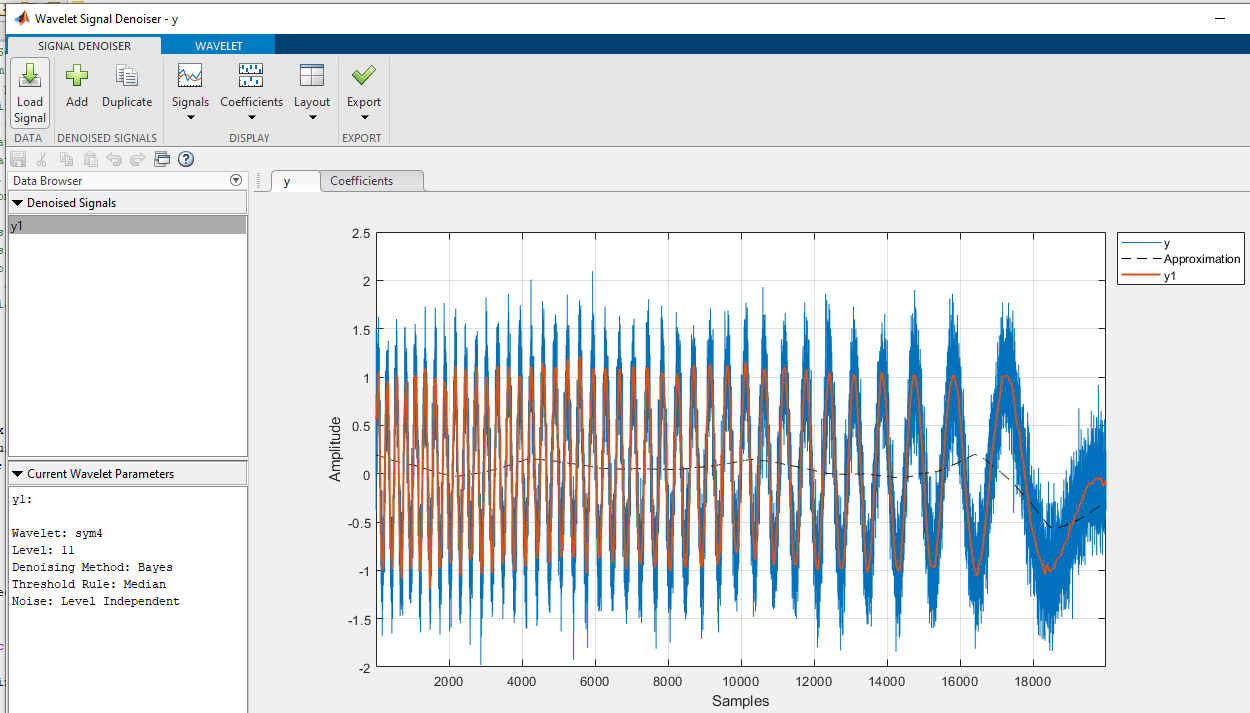So both the sym4 wavelet in the Denoiser and the Morlet wavelet’s time-frequency-amplitude matrix give evidence of the hidden Gaussian peaks, but displayed in a different way.

In addition to the GUI app, there is also a command-line function denioising function called “wdenoise.m” (syntax: wdenoise(noisydata,level,…). The selection of the wavelet type and level are set by including optional input arguments to this function. The advantage of using a function, compared to the GUI app, is that it’s possible to write scripts that quickly and automatically compare many different wavelet settings, that compare the results to several conventional noise reduction methods, or that automate the batch processing of large numbers of stored data sets (see Batch processing).  For example, the question of the optimal selection of wavelet level can be answered by the script OptimizationOfWaveletLevel3peaks.m, which creates a signal consisting of three noisy unit-height Gaussian (or Lorentzian) peaks with different peak widths, with added white noise.

The script uses the wdenoise.m function to denoise them with the “coiflet” wavelet from levels 1 to 11, measuring three quantities for each denoised signal: the height of the peaks, the signal-to-noise ratio improvement, and the closeness to the noiseless underlying signal, as shown in the three plots below.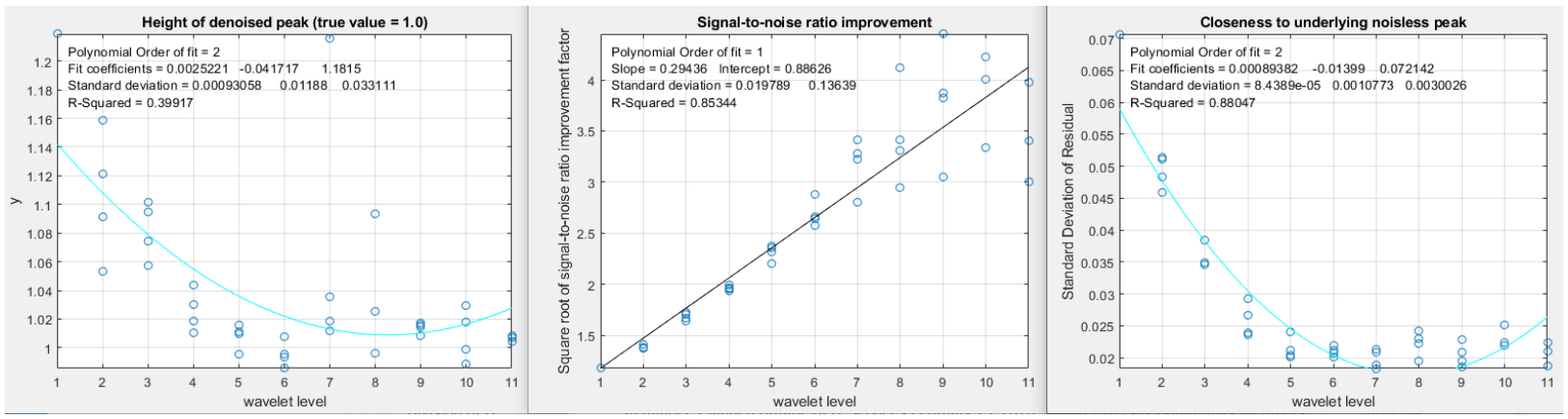We can see from these plots that a level of about 7 is optimum in this case; above 7, the signal-to-noise ratio (center graph) continues to increase but the results are unreliable and tend to scatter around too much. Changing to Lorentzian peaks (line 28 of the script) yields similar results.

The script WaveletsComparison.m compares five different wavelet types on the same signal: BlockJS, bior5.5, coif2, sym8, and db4, all at level 12 (graphic). The results are similar but the sym8 has a slight edge. For most smooth peak shapes with additive white noise, the different wavelets perform similarly. For signals with high-frequency weighted noise, the bior5.5 wavelet works better than the others (script; graphic). For square pulses, however, the Haar wavelet is clearly superior.

Another script compares five different non-wavelet smoothing techniques and two different wavelets, all using the same simulated signal consisting of two Gaussian peaks with a 50-fold difference in peak width, in order to test for adaptability to peak width variation. For each method, the percent errors in the peak height, width, and area are measured, as well as the “residual”, which is the difference between the underlying noiseless signal and the denoised (or smoothed) noisy signal. This illustrates a significant advantage that wavelet denoising has over smoothing; it adapts much better to changes in peak width. A summary of typical results is shown in this table and this graphic. (Peak 1 is the narrow peak and peak 2 is 50 times wider).

Typical results               Percent errors of peak1|peak2

Method        Residuals     Height         Width          Area

Original        9.88%    6.29%|25.8%    6.31%|-23.24%  -2.49%|0.86%

Gaussian        2.53%   -3.34%|-3.79%   5.72%|4.35%     -2.6%|0.82%

Segmented       2.04%  -24.48%|3.09%    37.8%|0.21%    -7.07%|0.85%

Savitsky-Golay  2.93%   -2.08%|6.45%    8.66%|-3.04%    -1.9%|0.86%

RC filter       3.29%   -6.53%|9.58%   16.06%|-5.45%  -11.76%|0.86%

Hamming filter  2.91%   -5.12%|8.7%     8.19%|-5.31%   -2.17%|0.86%

coif2 wavelet   1.02%    1.78%|1.18%   -5.59%|0.22%    -6.54%|0.75%

db2 wavelet     1.17%   11.47%|3.82%    3.36%|2.38%    -5.34%|0.81%

The “Residuals” are the percent differences between the underlying noiseless signal and the signal with random noise after denoising; it accounts for both residual noise left in the signal and distortion of the signal shape. As you can see, the coif wavelet comes out ahead by most measures. This illustrates the most significant practical advantages of wavelet denoising: (1) it gives results that are at least as good, and often better, than conventional smoothing methods, (2) it’s easier to use because it automatically adapts to different peak widths; and (3) it’s easier to optimize because usually only the level setting makes much difference.

However, there are a few situations where conventional methods are better. For example, in calculating the second derivatives of noisy peaks of variable width, a segmented Gaussian-weighted smooth (page 312) gives a signal-to-noise ratio better than that of a wavelet denoise (script; graphic), especially if the signal-to-noise ratio is poor (graphic), presumably because the frequency spectrum of the noise is so strongly high-frequency weighted. Also, wavelet denoising does not work if the amplitude of the noise is proportional to the signal amplitude rather than constant (script; graphic). Sometimes, if the original signal-to-ratio is very poor, wavelet denoising produces narrow spike artifacts in the denoised signals, even when soft thresholding is used. These are special cases; there are many more situations where the wavelet denoise is really the method of choice.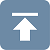Updated March, 2020. This page is part of "A Pragmatic Introduction to Signal Processing", created and maintained by Prof. Tom O'Haver, Department of Chemistry and Biochemistry, The University of Maryland at College Park. Comments, suggestions and questions should be directed to Prof. O'Haver at toh@umd.edu.
Unique visits since May 17, 2008: